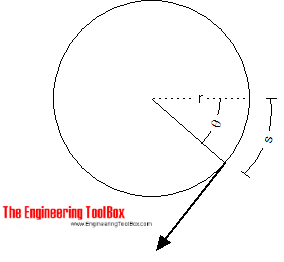Engineering ToolBox - Resources, Tools and Basic Information for Engineering and Design of Technical Applications!

# Torque - Work done and Power Transmitted

## The work done and power transmitted by a constant torque.

### Work done

Work done is the force multiplied with the distance moved by the force - and can be expressed as

W = F s                                  (1)

where

W = work done (J, Nm)

F = force (N)

s = distance moved by force (s)

For an angular motionthe work done can be expressed as

W = F θ r

= T θ                                     (2)

where

W = work (Joules)

T = torque or moment (Nm)

### Power transmitted

Power is the ratio between the work done and the time taken and can be expressed as

P = W / dt

= T θ / dt

= T ω

= 2 π n T

= 2 π (nrpm / 60) T

= 0.105 nrpm T                                  (3)

where

P = power (Watts)

dt = time taken (s)

ω =  θ / dt = 2 π n = angular velocity (rad/s)

n = speed (rev/s)

nrpm = speed (rev/min, rpm)

Note! - a machine must rotate to produce power! A machine with no rotation can deliver torque - like an electric motor - but since no distance is moved by force - no power is produced. As soon as the machine starts to rotate power is produced.

### Example - required Torque to produce Power

A machine rotates with speed 3000 rev/min (rpm) and consumes 5 kW. The torque at the shaft can be calculated by modifying (3) to

T = P / 2 π n

= (5 kW) (1000 W/kW) / 2 π (3000 rev/min) / (60 sec/min)

= 15.9 Nm

## Related Topics

• ### Mechanics

Forces, acceleration, displacement, vectors, motion, momentum, energy of objects and more.

## Related Documents

• ### Belt Transmissions - Speed and Length of Belts

Calculate length and speed of belt and belt gearing.
• ### Belts - Power Transmission and Efficiency

Calculate belts power transmission and efficiency
• ### Conn-Rod Mechanism

The connecting rod mechanism.
• ### Distance Traveled vs. Speed and Time - Calculator and Chart

Velocity plotted in time used diagram.
• ### Electric Motors - IEC and NEMA Standard Torques

IEC and NEMA torque classifications of electrical motors.
• ### Electric Motors - Torque vs. Power and Speed

Electric motor output power and torque vs. rotation speed.
• ### Electrical Induction Motors - Torque vs. Speed

Full load operating torque vs. break down, pull up and locked rotor torque.
• ### Elevators - Force and Power

Required force and power to lift an elevator.
• ### Improvised Torque Wrench

Improvise a torque wrench with a luggage scale.
• ### Mass Moment of Inertia

The Mass Moment of Inertia vs. mass of object, it's shape and relative point of rotation - the Radius of Gyration.
• ### Power

Power is the rate at which work is done or energy converted.
• ### Rotating Shafts - Torque

Torsional moments acting on rotating shafts.
• ### Shafts Torsion

The torsion of solid or hollow shafts - Polar Moment of Inertia of Area.
• ### Torque or Moment of Force - Online Converter

Torque or moment - the tendency of a force to rotate an object.
• ### Work done by Force

Work done by a force acting on an object.

## Engineering ToolBox - SketchUp Extension - Online 3D modeling!

Add standard and customized parametric components - like flange beams, lumbers, piping, stairs and more - to your Sketchup model with the Engineering ToolBox - SketchUp Extension - enabled for use with older versions of the amazing SketchUp Make and the newer "up to date" SketchUp Pro . Add the Engineering ToolBox extension to your SketchUp Make/Pro from the Extension Warehouse !

We don't collect information from our users. More about

## Citation

• The Engineering ToolBox (2008). Torque - Work done and Power Transmitted. [online] Available at: https://www.engineeringtoolbox.com/work-torque-d_1377.html [Accessed Day Month Year].

Modify the access date according your visit.

9.29.12